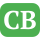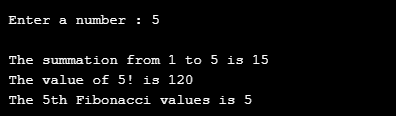CppBuzz.com

# C program to print sum, factorial & fibonacci series

This C program take any number as input and then prints its sum ( 1 to Number ), its factorial and last digit of fibonacci series. It doesn't work for negative number.

``````#include<stdio.h>

int summation(int);
int factorial(int);
int FibonacciNthNo(int);

int main(){
int no;
printf("\n Enter a number : ");
scanf("%d",&no);

if(no<0){
printf("\n Input value should be a positive integer");
return -1;
}

printf("\n The summation from 1 to %d is %d",no, summation(no));
printf("\n The value of %d! is %d",no, factorial(no));
printf("\n The %dth Fibonacci values is %d",no, FibonacciNthNo(no));

return 0;
}

int summation(int no){
int sum = 0;
for(int i=1; i<=no; i++)
sum += i;
return sum;
}

int factorial(int no){
int fact = 1;
int temp = no;
while(temp>0){
fact *= temp--;
}

return fact;
}

int FibonacciNthNo(int no){
int a,b,i,temp;
i = a= b = 1;

/*
remove this comment if you want to print Fibonacci sercies
printf("%d %d ",a,b);
*/

do{
temp = a+b;
a=b;
b=temp;

/*
remove this comment if you want to print Fibonacci sercies
printf("%d ",temp);
*/
}while(i++<no-2);

return b;
}
``````